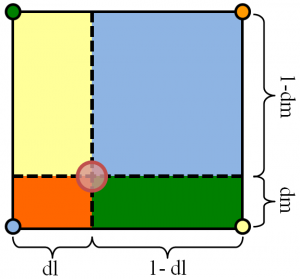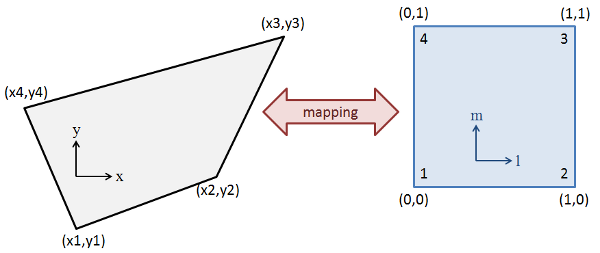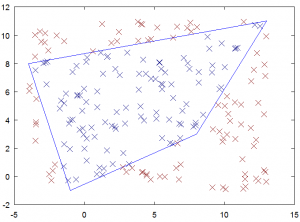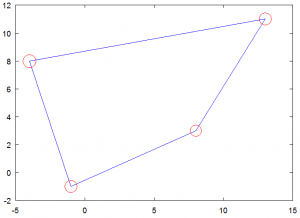Interpolation using an arbitrary quadrilateral

Posted on June 4th, 2012
Previous Article :: Next Article

In the previous article on the Particle In Cell method (PIC), we introduced the concept of scattering data to the grid. The PIC method relies heavily on the interpolation of data between particles and the grid. First, the scatter operation is used to compute charge density which is then fed to the Poisson solver. The computed electric field, which is stored on grid nodes, is subsequently gathered back onto the particle positions to calculate their acceleration.

Mesh interpolation

The interpolation technique we used in that example is based on linear area weighing. There are other alternatives (see  for instance). Some, such as the nearest point method, are less accurate. Others utilize second or higher order scheme to increase accuracy. The linear method described here offers a good compromise between accuracy and the computational overhead. This is the method that is used in all our codes.

This interpolation scheme is summarized below in Figure 1. We want to interpolate some quantity existing at the position denoted by the large red circle to the four grid nodes. To do this, we divide the cell into four sections (or eight in 3D). The fraction to be deposited to each node is simply given by the ratio of the area diagonally opposite from the node to the total cell area, $$w_i=A_i/A$$ (in 3D you would use volume ratios). To help you visualize this, in Figure 1, the nodes are shaded with the color corresponding to the area fraction that gets deposited to it.Figure 1. Computational cell is subdivided and fraction corresponding to the diagonal area is deposited onto each node.

When making this calculation it helps to work in logical (or natural) coordinates. These are coordinates that define the position in the cell as a fraction of the cell distance along the corresponding dimension. In this article we label these coordinates as $$l=[0,1]$$ and $$m=[0,1]$$. Value of zero corresponds to the left or bottom edge, and value of 1 is the right or top edge. For a Cartesian system such as the one used in the example PIC code we can easily obtain these coordinates as $$i_f=(x-x_0)/\Delta x$$ and $$l=i_f-\text{int}(i_f)$$ (the “f” subscript was added to indicate that $$i_f$$ is a floating point number and not an integer). In logical coordinates the total area is unity, and thus we simply need to multiply the value to be scattered by the corresponding sectional area. The respective lengths are called out in Figure 1. To gather from the grid to the particle we simply multiply the value at each node by the opposite area and add the contributions together, $$n_x = w_1n_1 + w_2n_2 + w_3n_3 + w_4n_4$$.

Mapping function for an arbitrary quadrilateral

The above technique works but it has one serious drawback: as written it is useful only for rectangular cells. But often we prefer to use cells that are irregular. Even if we do not use the finite element method, we may want to use method such as finite volume along with cut cells to approximate the surface boundary. Or we may want to use a body-fitted structured mesh. These methods will give us mesh cells that are no longer rectilinear but are arbitrary quadrilaterals. Of course, you could end up with different shapes such as triangles or three dimensional wedges depending on your setup, but here for simplicity we consider only 2D quads. Ref  has a good overview of various cell types.

In order to perform the interpolation on an arbitrary quad, we need to obtain a mapping function as shown in Figure 2. Our goal is to come up with a functions such as $$(x,y)=f(l,m)$$ where $$l=[0,1]$$ and $$m=[0,1]$$ describes the entire point space enclosed by the quadrilateral. In addition, we want $$f(0,0)=(x_1,y_1)$$, $$f(1,0)=(x_2,y_2)$$ and so on to correspond to the polygon vertices. This function, yet to be determined, forms a map that allows us to transform the quad from the physical coordinate set to a logical coordinate space. In the logical coordinates, the polygon morphs into a square, regardless of its physical form. Once the logical coordinates are obtained, we perform the scatter and gather operations just as described in the above paragraph.Figure 2. A mapping function allows us to represent the arbitrary shaped quadrilateral as a square

What remains now is finding the map. To do this, we assume a bilinear mapping function  given by
$$x=\alpha_1 + \alpha_2 l + \alpha_3 m + \alpha_4 lm$$
and
$$y=\beta_1 + \beta_2 l + \beta_3 m + \beta_4 lm$$
We next use these expressions to solve for the four coefficients,
$$\left[\begin{array}{c}x_1\\x_2\\x_3\\x_4\end{array}\right] = \left[\begin{array}{cccc}1&0&0&0\\1&1&0&0\\1&1&1&1\\1&0&1&0\end{array}\right] \left[\begin{array}{c}\alpha_1\\\alpha_2\\\alpha_3\\\alpha_4\end{array}\right]$$
The coefficients in the matrix come from Figure 2. For instance for node “1” we have (l,m)=(0,0). A similar expression is also written for the betas. We can solve for the coefficients easily by inverting the matrix,
$$\left[\begin{array}{c}\alpha_1\\\alpha_2\\\alpha_3\\\alpha_4\end{array}\right] = \left[\begin{array}{cccc}1&0&0&0\\-1&1&0&0\\-1&0&0&1\\1&-1&1&-1\end{array}\right] \left[\begin{array}{c}x_1\\x_2\\x_3\\x_4\end{array}\right]$$

Using Matlab syntax, we can write

%create our polygon
px = [-1, 8, 13, -4];
py = [-1, 3, 11, 8];

%compute coefficients
A=[1 0 0 0;1 1 0 0;1 1 1 1;1 0 1 0];
AI = inv(A);
a = AI*px';
b = AI*py';

We now have our mapping to go from the logical to the physical world. To obtain the reverse mapping we need to solve
$$\begin{array}{rcl} x&=&\alpha_1 + \alpha_2l +\alpha_3m+\alpha_4 lm \\ y&=&\beta_1 + \beta_2l +\beta_3m+\beta_4 lm\end{array}$$
Note this system is no longer linear, however it can be solved quite easily analytically. You can first obtain
$$l= \left(\dfrac{x-\alpha_1-\alpha_3 m}{\alpha_2+\alpha_4 m}\right)$$
which can then be substituted into the second expression (this is as they say, “an exercise left for the reader”) to obtain
$$(\alpha_4\beta_3 – \alpha_3\beta_4)m^2 + (\alpha_4\beta_1-\alpha_1\beta_4+\alpha_2\beta_3 -\alpha_3\beta_2 + x\beta_4 – y\alpha_4)m + (\alpha_2\beta_1 – \alpha_1\beta_2 + x\beta_2 – y\alpha_2)=0$$
This is simply a quadratic equation with the three coefficients given by the terms in the parentheses and with the physical solution $$m = (-b + \sqrt{b^2-4ac})/2a$$. The Matlab/Octave function below does this calculation and converts the supplied physical coordinates to logical ones using the also-supplied coefficients.

% converts physical (x,y) to logical (l,m)
function [l, m] = XtoL(x,y,a,b)
%quadratic equation coeffs, aa*mm^2+bb*m+cc=0
aa = a(4)*b(3) - a(3)*b(4);
bb = a(4)*b(1) -a(1)*b(4) + a(2)*b(3) - a(3)*b(2) + x*b(4) - y*a(4);
cc = a(2)*b(1) -a(1)*b(2) + x*b(2) - y*a(2);

%compute m = (-b+sqrt(b^2-4ac))/(2a)
det = sqrt(bb*bb - 4*aa*cc);
m = (-bb+det)/(2*aa);

%compute l
l = (x-a(1)-a(3)*m)/(a(2)+a(4)*m);
end

Example 1: Picking random points in a quadrilateral

Now we have everything we need to have some fun. The first example shows how to pick random points that are located within a prescribed quad. Any point located within the polygon will have natural coordinates in the 0 to 1 range. Hence we just need to pick two random natural coordinates and convert them with the mapping function. The code is given below. The real part is the part in the loop – the second half is simply a code to generate the plot. You will get a plot similar to the one in Figure 3, however the actual point locations will vary. Notice that all points are located within the polygon bounds.

%plots random points inside the polygon
function []=plot_points_in_poly(px,py,a,b)

%pick random logical coordinate and convert to physical
for i=1:100
l=rand();
m=rand();
x(i) = a(1) + a(2)*l + a(3)*m + a(4)*l*m;
y(i) = b(1) + b(2)*l + b(3)*m + b(4)*l*m;
end

%plot
figure(1);
hold off;
plot([px px(1)],[py py(1)]);
hold on;
plot (x,y,'x');
end

Example 2: Determining if a point lies inside or outside the quadrilateral

The first example showed how to go from the logical to the physical coordinates. The second example is the opposite. In this example we pick 200 points randomly distributed in the polygon bounding box and determine if they are inside or outside the polygon. To accomplish this, we use the fact that a point inside the polygon will have both natural coordinates in the 0 to 1 range. Although here we simply flag the node location, a variant of this technique could be used in an unstructured code to search for the cell containing a particle. A coordinate $$m>1$$ indicates that the next searched cell should be the one sharing the $$m=1$$ edge (whatever this means physically depends on the mapping). This is much faster than looping through the cells one by one. When you run the code below, you will get a plot similar to the one in Figure 4

%picks random points and colors them based on internal or external
function []=plot_internal_and_external_points(px,py,a,b)

%bounding box
x0 = min(px);
lx = max(px)-min(px);
y0 = min(py);
ly = max(py)-min(py);

%convert random physical coordinates to logical
for i=1:200

%pick a random point in the bounding box
x(i) = x0+rand()*lx;
y(i) = y0+rand()*ly;

%calculate logical coordinates
[l,m] = XtoL(x(i),y(i),a,b);

%is the point outside the quad?
if (m<0 || m>1 || l<0 || l>1)
type(i)=1;
else
type(i)=0;
end
end

%plot
figure(2);
hold off;
plot([px px(1)],[py py(1)]);
hold on;
scatter(x,y,4,type,'x');
endFigure 4. Points randomly sampled in the polygon bounding box and classified by their location.

Example 3: Scattering data to polygon nodes

Next we consider something of actual use to the PIC method: the scatter operation. Here we pick 100 random points inside the polygon and scatter the value of “1” for each point. This in essence counts the number of points in the polygon. Given a large number of samples and a good random number generator, we should get the value of 25 at each node. In the code below we scale this data such that the expected value on each node is 10 (this was done for visualization purposes). When I ran the code below I got:
9.8026 9.6121 9.8507 10.7346
These values add up to 40 as expected. Figure 5 shows this data graphically with the size of the circle corresponding to the node value. If the density was not uniform, we would see the circles have varying sizes.

%scatters counts to the four grid nodes
function []=scatter_data(px,py,a,b)

%init
c = [0 0 0 0];

%pick random logical coordinate and convert to physical
for i=1:100

%random point inside the quad
l=rand();
m=rand();

%deposit counts, note here l,m=[0:1]. In a real 2d mesh
%the index we would first need to subtract the integral
%cell index to get the cell-local distance, dl = l - (int)l;
dl = l;
dm = m;

c(1) += (1-dl)*(1-dm);
c(2) += dl *(1-dm);
c(3) += dl * dm;
c(4) += (1-dl)*dm;
end

%scale data, uniform distribution = 10
c = 40*(c/i);

%output
disp(c);

%plot
figure(3);
hold off;
plot([px px(1)],[py py(1)]);
hold on;
scatter(px,py,c,[1 0 0], 'o');
endFigure 5. Counts for particles sampled inside the polygon are scattered to the polygon nodes resulting in uniform distribution.

Example 4: Gathering data from polygon nodes onto a particle

And finally, here is the opposite of the previous example. Here we interpolate data from the grid nodes onto the particle position. This is analogous to gathering electric field components prior to evaluating acceleration. In this example we set the values [1,2,3,4] onto the polygon vertices. To better illustrate this interpolation, we construct a 30×15 uniform grid and interpolate the polygon values onto the grid points. We only do this for points located inside the polygon. The 2D grid allows us to visualize the results as a contour flood. The example starts by constructing the grid. It then loops through the grid nodes and performs the calculation using each grid node position. The output is shown in Figure 6.

%interpolates data from the polygon vertices onto a grid
function []=gather_data(px,py,a,b)

%bounding box
x0 = min(px);
lx = max(px)-min(px);
y0 = min(py);
ly = max(py)-min(py);

%set spacing such that we have 30x30 grid (i.e. 29 cells)
ni = 30;
nj = 15;

dx = lx/(ni-1);
dy = ly/(nj-1);

%node positions (for plotting)
for i=1:ni
x(i) = x0 + (i-1)*dx;
end
for j=1:nj
y(j) = y0 + (j-1)*dy;
end

%node values
c = [1 2 3 4];

val=zeros(nj,ni);

for i=1:ni
for j=1:nj
%convert to logical coordinates
[l m] = XtoL(x(i),y(j),a,b);

%evaluate only if we are inside
if (l>0 && l<=1 && m>=0 && m<=1)

%again, if we had multiple cells, we would set dl = l - (int)l
dl = l;
dm = m;

val(j,i) = (1-dl)*(1-dm)*c(1) + ...
dl*(1-dm)*c(2) + ...
dl*dm*c(3) +...
(1-dl)*dm*c(4);
end
end
end

%plot
figure(4);
hold off;
contourf(x,y,val);
hold on;
plot([px px(1)],[py py(1)],'w','LineWidth',4);
end

Complete Source Code

You can get the complete source code here: interpolate2d.m.

As an aside, this code was tested using Octave 3.2.4, and not Matlab, since I do not have a copy of the latter. Octave is a free open source Matlab emulator than can be downloaded from octave.org or as a Cygwin package. The Cygwin version is newer (version 3.6.2 as of writing) but since it requires few additional steps such as (1) installing Cygwin and (2) installing XWin I used the older (and quite buggy) pre-built Windows version from the Octave site.

References

 Birdsall, C. K., and Langdon, A.B., “Plasma Physics Via Computer Simulations“, Institute of Physics Publishing, 2000

 Hughes, T. J. R., “The Finite Element Method“, Dover Publications, 2000

Subscribe to the newsletter and follow us on Twitter. Send us an email if you have any questions.

23 comments to “Interpolation using an arbitrary quadrilateral”

1. Larry Stimac
October 19, 2012 at 7:40 am

helped a lot with matching ground projections…thanks

• October 29, 2012 at 9:55 am

Great, glad it helped!

2. Larry Stimac
October 19, 2012 at 9:01 am

In xtol I found had to check the coefficient on the m^2 term for zero and if so solve the linear equation. If the quad is square this happens.

3. Kevin Raeder
February 18, 2014 at 10:07 am

Thanks for this web site! I found it very useful for identifying the quadrilateral cell on a sphere which contains an arbitrary location. I’m puzzled, though, by one part o the development, above. The ‘+’ root of the quadratic equation for m is chosen as the ‘physical’ solution. I can understand that if the b coefficient is never negative, but I can’t convince myself that that is true. Can you provide a longer explanation of that choice?
Thanks,
Kevin

4. Zhiyuan Pan
January 24, 2015 at 6:13 am

The method is not always working, possibly when two points on x or y axis, or very close to. The following is a failure case.
(x1,y1) = (2.31, 0)
(x2,y2) = (2.2, 0)
(x3,y3) = (2.12, 1.8)
(x4,y4) = (2.24, 1.9)
A point (2.17, 0.62) should be inside the quar panel. However, the proposed method gives interpolated coordinate as (13.05,-6.63)
Could you please check why, and any proposal to cover it up?

• Alex
June 10, 2015 at 8:55 pm

I think your 5th point is outside the quadrilateral.
The method is generally correct.

5. Alex
June 10, 2015 at 8:56 pm

m = (-b + sqrt{b^2-4ac})/2a.

in my case I need to use
m = (-b – sqrt{b^2-4ac})/2a. to get the m and l to be within range of [0 1]

• June 12, 2015 at 3:34 pm

Thanks. I think there is some logic missing because it seems that in some cases the “+” is needed and in others, it’s the “-“.

6. Peter
March 30, 2016 at 6:03 am

The analytical solution works only when a2 + a4m != 0, which is not always the case.

• Pavlo
November 30, 2017 at 3:22 am

The problem could arise when the point (x2,y2) is lower then (x1,y1). This could be solved by rotating the polygon by 90 degrees in clockwise direction. Literally you should change the points order from (1, 2, 3, 4) to (4, 1, 2, 3).
I have had the same problem and the solution works for me fine.

7. Christian
July 5, 2016 at 7:43 am

Does this method break down when used for a rectangle? I have tried the following points and it did not work:

px = [-18.0802 -18.0602 -18.0602 -18.0802];
py = [-27.5042 -27.5042 -27.4042 -27.4042];

and trying to interpolate the point

x = -18.0627
y=-27.4659

• July 20, 2016 at 7:56 am

I noticed there are some cases for which the method does not work. I suppose it could be due to $$\alpha_2$$ and $$\alpha_4$$ being zero, which would then make $$l$$ undefined. I think in those cases you need to use an expression for $$m$$ and using it to solve for $$m$$ (basically the opposite of what is done here). I did not derive that form of the equations yet but you may want to try to see if that helps with this rectangle.

8. Alexis Farmer
July 31, 2016 at 10:08 pm

It just needs to be fixed for the case where aa is equal to zero, which leads to a divide by zero. I’m not using matlab but basically in a C/Java like syntax then

if ( aa == 0.0 )
m = -cc/bb;

Otherwise I was getting m to be infinite

9. Alexis Farmer
August 1, 2016 at 8:42 am

Ok, the other case when it doesn not work is when the arbritrary quadrilateral is in the region of 90 degrees rotated from the unit square. To be honest I have not found a solution for this yet.

10. Clocktown
December 12, 2016 at 4:51 pm

I implemented this for my QuadLights in my C++ Raytracer, for generating random sampling positions (for SoftShadows). I extended it because the points on my QuadLight are obviously in 3D-Space (not 2D), but all lie on a plane.

I have one question though: Does this work with concave Quadriliterals?
I’d test it myself but right now my time is limited so I’m not sure when I’ll get to that.

• December 19, 2016 at 8:36 am

Re concave quads: I have not yet tested that but my guess would be likely not.

11. Waterman
April 30, 2017 at 6:56 pm

Quadrilateral physical -> logical coordinates was great help for aligning ortho images! Thx a lot!

12. abc
December 10, 2018 at 1:08 pm

What does it mean if sqrt(b^2-4*a*c) is negative?

• abc
December 10, 2018 at 1:09 pm

of course I mean if the term in the square root is negative

• abc
December 10, 2018 at 2:14 pm

to give an example, for the convex quadrangle with the counterclockwise coordinates
(x1,y1)=(2.3,8.04)
(x2,y2)=(2.8,7.8)
(x3,y3)=(2.8,8.99)
(x4,y4)=(1.9,9.03)
and the a test point that lies outside of the quadrangle
(x,y)=(1,3)
the term b^2-4ac becomes negative, exactly -0.748

13. Jo
February 5, 2019 at 7:43 am

Thank you! This was the most comprehensive, and above all *IMPLEMENTABLE* (in C/C++/C#/Java etc) solutions to convex quadrilateral mapping onto the unit square that I’ve found. Many articles out there are too math oriented with vectors and matrixes that they are hard to solve analytically as simply as you have shown. Some are even plain wrong (requiring a paralellogram for the solution to work), but this works as a charm! It’s also easy to detect when resulting real world values are not within the quad and thus the unit square as some results become complex or negative.
Thanks!

• February 15, 2019 at 7:16 pm

Thanks!

14. June 9, 2019 at 4:12 am

I faced a similar problem. I was working with weather data (wind direction and speed) for altitude layers. I was trying to determine the drop point to get an object to land at a given point. I used the data over the landing point to determine the drop point 5000 feet above the altitude of the landing point. Then subsequently up in altitude in 5000 foot incriments. This meant that I had to calculate an average for calculated points that were the end vectors within each grid the dropped object fell. I concluded that the values I should assign could be determined by calculating from the larger grid baised on an inverse percentage of the areas defined by a rectangle divided by the calculated point.
like the diagram at